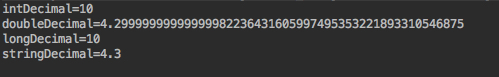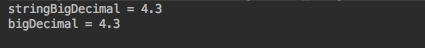# BigDecimal详解和精度问题

## 一、背景

1、double计算会有精度丢失问题

2、在除法运算时，BigDecimal提供了丰富的取舍规则。(double虽然可以通过NumberFormat进行四舍五入，但是NumberFormat是线程不安全的)

  public static void main(String[] args) {
//正常 3.3
System.out.println("加法结果："+(1.1+2.2));
//正常 -7.9
System.out.println("减法结果："+(2.2-10.1));
//正常 2.42
System.out.println("乘法结果："+(1.1*2.2));
//正常 0.44
System.out.println("除法结果："+(4.4/10));
}为什么会这样原因？

## 二、BigDecimal构造函数

#### 1、四种构造函数

BigDecimal(int)     //创建一个具有参数所指定整数值的对象。
BigDecimal(double)  //创建一个具有参数所指定双精度值的对象。
BigDecimal(long)    //创建一个具有参数所指定长整数值的对象。
BigDecimal(String)  //创建一个具有参数所指定以字符串表示的数值的对象。


toString()          //将BigDecimal对象的数值转换成字符串。
doubleValue()       //将BigDecimal对象中的值以双精度数返回。
floatValue()        //将BigDecimal对象中的值以单精度数返回。
longValue()         //将BigDecimal对象中的值以长整数返回。
intValue()          //将BigDecimal对象中的值以整数返回。


public static void main(String[] args) {
BigDecimal intDecimal = new BigDecimal(10);
BigDecimal doubleDecimal = new BigDecimal(4.3);
BigDecimal longDecimal = new BigDecimal(10L);
BigDecimal stringDecimal = new BigDecimal("4.3");
System.out.println("intDecimal=" + intDecimal);
System.out.println("doubleDecimal=" + doubleDecimal);
System.out.println("longDecimal=" + longDecimal);
System.out.println("stringDecimal=" + stringDecimal);
}#### 2、为什么会出现这种情况

0.1000000000000000055511151231257827021181583404541015625。 这是因为0.1不能像double （或者作为任何有限长度的二进制分数）精确地表示。

#### 3、如何解决

1、是将double 通过Double.toString(double)先转为String，然后放入BigDecimal的String构造函数中。

2、不通过BigDecimal的构造函数，而是通过它的静态方法BigDecimal.valueOf(double),也同样不会丢失精度。

示例

 public static void main(String[] args) {
String string = Double.toString(4.3);
BigDecimal stringBigDecimal = new BigDecimal(string);
BigDecimal bigDecimal = BigDecimal.valueOf(4.3);
System.out.println("stringBigDecimal = " + stringBigDecimal);
System.out.println("bigDecimal = " + bigDecimal);
}## 三、常用方法

#### 1、常用方法

示例

public static void main(String[] args) {
BigDecimal a = new BigDecimal("4.5");
BigDecimal b = new BigDecimal("1.5");
BigDecimal c = new BigDecimal("-10.5");

BigDecimal subtract_result = a.subtract(b);
BigDecimal multiply_result = a.multiply(b);
BigDecimal divide_result = a.divide(b);
BigDecimal remainder_result = a.remainder(b);
BigDecimal max_result = a.max(b);
BigDecimal min_result = a.min(b);
BigDecimal abs_result = c.abs();
BigDecimal negate_result = a.negate();

System.out.println("4.5-1.5=" + subtract_result);
System.out.println("4.5*1.5=" + multiply_result);
System.out.println("4.5/1.5=" + divide_result);
System.out.println("4.5/1.5余数=" + remainder_result);
System.out.println("4.5和1.5最大数=" + max_result);
System.out.println("4.5和1.5最小数=" + min_result);
System.out.println("-10.5的绝对值=" + abs_result);
System.out.println("4.5的相反数=" + negate_result);
}


运行结果

4.5+1.5=6.0
4.5-1.5=3.0
4.5*1.5=6.75
4.5/1.5=3
4.5/1.5余数=0.0
4.5和1.5最大数=4.5
4.5和1.5最小数=1.5
-10.5的绝对值=10.5
4.5的相反数=-4.5


no exact representable decimal result。所以这里要考虑除不尽的情况下，保留几位小数,取舍规则。（除法如果可能存在除不进，那就用下面方法）

BigDecimal divide(BigDecimal divisor, int scale, int roundingMode) 第一参数表示除数，第二个参数表示小数点后保留位数，第三个参数表示取舍规则。


#### 2、取舍规则

ROUND_UP          //不管保留数字后面是大是小(0除外)都会进1
ROUND_DOWN        //保留设置数字，后面所有直接去除
ROUND_HALF_UP     //常用的四舍五入
ROUND_HALF_DOWN   //五舍六入
ROUND_CEILING     //向正无穷方向舍入
ROUND_FLOOR       //向负无穷方向舍入
ROUND_HALF_EVEN   //向（距离）最近的一边舍入，除非两边（的距离）是相等,如果是这样，如果保留位数是奇数，使用ROUND_HALF_UP，如果是偶数，使用ROUND_HALF_DOWN
ROUND_UNNECESSARY //计算结果是精确的，不需要舍入模式


注意 我们最常用的应该是 ROUND_HALF_UP(四舍五入)

 public static void main(String[] args) {

BigDecimal a = new BigDecimal("1.15");
BigDecimal b = new BigDecimal("1");

//不管保留数字后面是大是小(0除外)都会进1 所以这里输出为1.2
BigDecimal divide_1 = a.divide(b,1,BigDecimal.ROUND_UP);
//保留设置数字，后面所有直接去除         所以这里输出为1.1
BigDecimal divide_2 = a.divide(b,1,BigDecimal.ROUND_DOWN);
//常用的四舍五入         所以这里输出1.2
BigDecimal divide_3 = a.divide(b,1,BigDecimal.ROUND_HALF_UP);
//这个可以理解成五舍六入   所以这里输出1.1
BigDecimal divide_4 = a.divide(b,1,BigDecimal.ROUND_HALF_DOWN);
//这里将1.15改成1.16
BigDecimal c = new BigDecimal("1.16");
//那么这里就符合六入了 所以输出变为1.2
BigDecimal divide_5 = c.divide(b,1,BigDecimal.ROUND_HALF_DOWN);
System.out.println("divide_1 = " + divide_1);
System.out.println("divide_2 = " + divide_2);
System.out.println("divide_3 = " + divide_3);
System.out.println("divide_4 = " + divide_4);
System.out.println("divide_5 = " + divide_5);

}


运行结果

divide_1 = 1.2
divide_2 = 1.1
divide_3 = 1.2
divide_4 = 1.1
divide_5 = 1.2


## 四、格式化

BigDecimal对象作为format()方法的参数，输出其格式化的货币值和百分比。

示例

    public static void main(String[] args) {
//建立货币格式化引用
NumberFormat currency = NumberFormat.getCurrencyInstance();
//建立百分比格式化引用
NumberFormat percent = NumberFormat.getPercentInstance();
//百分比小数点最多3位
percent.setMaximumFractionDigits(3);
//取整
NumberFormat integerInstance = NumberFormat.getIntegerInstance();
////金额
BigDecimal loanAmount = new BigDecimal("188.555");
////利率
BigDecimal interestRate = new BigDecimal("0.018555555");
//没有指定保留位数的情况下 默认保留2位
System.out.println("金额: " + currency.format(loanAmount));
//货币(百分比)格式化   指定默认的取舍规则是四舍五入
System.out.println("利率: " + percent.format(interestRate));
//取整还有点不一样 188.555取整为189, 188.51也是189 但是189.5确实188,所以它不是真正意义上的四舍五入
System.out.println("取整: " + integerInstance.format(loanAmount));
}


运行结果

金额: ￥188.56



1、格式化的时候没有指定保留位数的情况下 默认保留2位

2、货币(百分比)格式化 指定默认的取舍规则是四舍五入

3、取整还有点不一样 188.555取整为189, 188.51也是189 但是189.5确实188,所以它不是真正意义上的四舍五入

别人骂我胖，我会生气，因为我心里承认了我胖。别人说我矮，我就会觉得好笑，因为我心里知道我不可能矮。这就是我们为什么会对别人的攻击生气。


posted on 2020-08-03 22:14  雨点的名字  阅读(174)  评论(0编辑  收藏